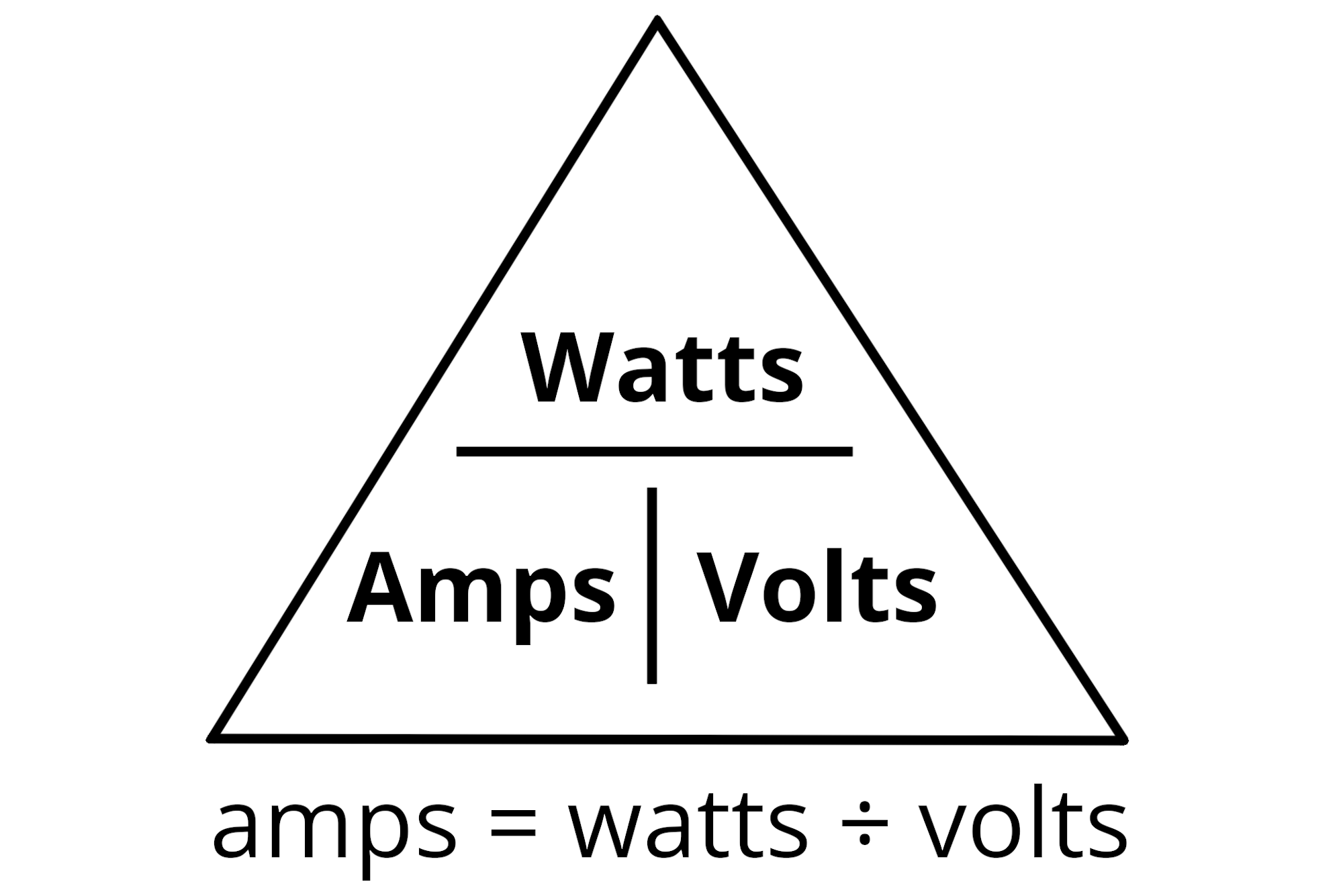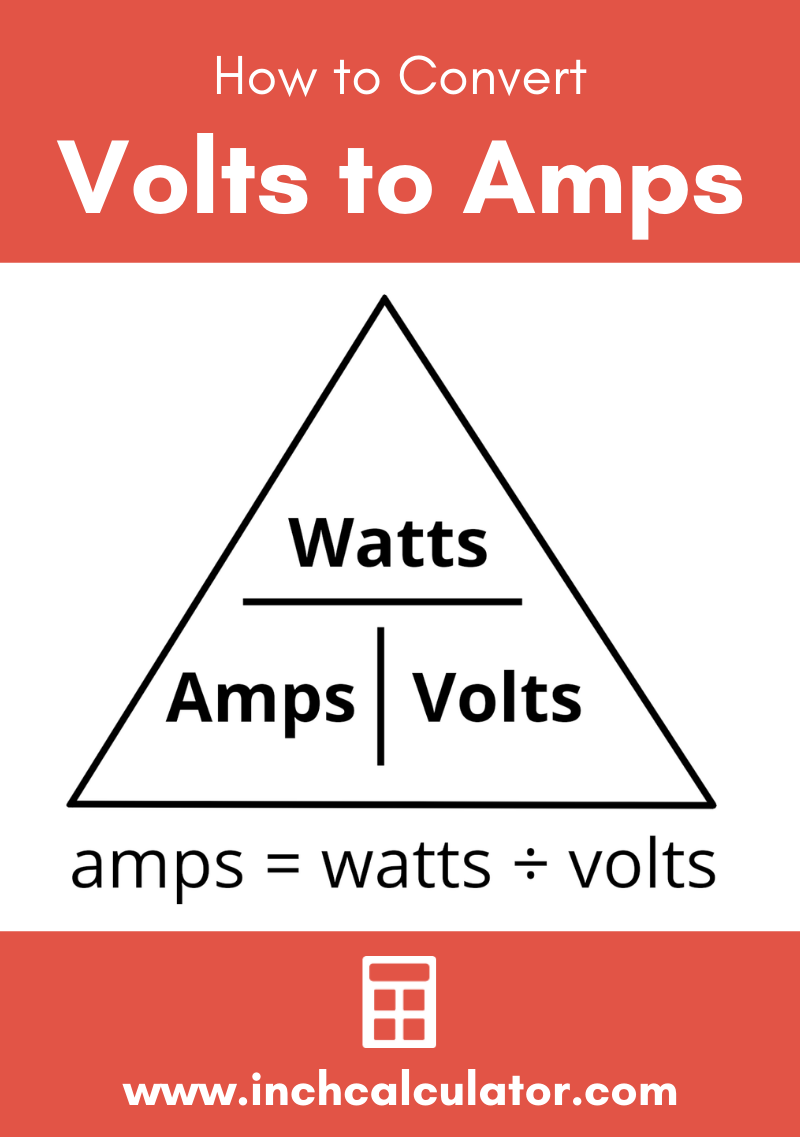# Volts to Amps Conversion Calculator

Convert volts to amps by entering the voltage and the electrical power in watts, or the resistance of the circuit.

## Current:

 Amps Milliamps
Learn how we calculated this below

## How to Convert Volts to Amps

Voltage is the electric potential difference in an electrical circuit, measured in volts. It might be easier to think of it as the amount of force or pressure pushing electrons through a conductor.

Electric current is a measure of the flow of electrons in a circuit, and is often measured in amperes.

Since these are different quantities or properties, to convert volts to amps, you need to use the Watt’s Law power formula or the Ohm’s Law formula.

The Watt’s Law power formula states that current = power ÷ voltage. Power is measured in watts and voltage is measured in volts. In AC circuits, use the RMS voltage as input and the output will be in RMS amps.

### Volts to Amps Formula

Thus, to find amps substitute the volts and watts in the formula:

I(A) = P(W) / V(V)

So, the current I in amps is equal to the power P in watts divided by the voltage V in volts.For example, let’s calculate the amperage of a 100-watt light bulb at 120 volts.

amps = 100 W / 120 V
amps = 0.83 A

A 100-watt light bulb at 120 volts will draw 0.83 amps of current.

## Convert Volts to Amps using Resistance

Ohm’s Law provides an alternate formula to find volts if amps and the electrical resistance are known. To calculate amps, divide the voltage by the resistance in ohms.

I(A) = V(V) / R(Ω)

The Ohm’s Law formula states that the current I in amps is equal to the voltage V in volts divided by the resistance R in ohms.

For example, let’s find the current of a 12-volt circuit with 10 ohms of resistance.

amps = 12 V / 10 Ω
amps = 1.2 A

A 12-volt circuit with a resistance of 10 ohms will draw 1.2 amps of current.

## Volts to Amps Chart

The table below shows volts converted to amps at various power ratings and RMS voltages.

Chart showing volts converted to amps at various power ratings.
Voltage Current Power
5 Volts 1 Amp 5 Watts
5 Volts 2 Amps 10 Watts
5 Volts 3 Amps 15 Watts
5 Volts 4 Amps 20 Watts
5 Volts 5 Amps 25 Watts
5 Volts 6 Amps 30 Watts
5 Volts 7 Amps 35 Watts
5 Volts 8 Amps 40 Watts
5 Volts 9 Amps 45 Watts
5 Volts 10 Amps 50 Watts
5 Volts 11 Amps 55 Watts
5 Volts 12 Amps 60 Watts
5 Volts 13 Amps 65 Watts
5 Volts 14 Amps 70 Watts
5 Volts 15 Amps 75 Watts
5 Volts 16 Amps 80 Watts
5 Volts 17 Amps 85 Watts
5 Volts 18 Amps 90 Watts
5 Volts 19 Amps 95 Watts
5 Volts 20 Amps 100 Watts
12 Volts 0.4167 Amps 5 Watts
12 Volts 0.8333 Amps 10 Watts
12 Volts 1.25 Amps 15 Watts
12 Volts 1.667 Amps 20 Watts
12 Volts 2.083 Amps 25 Watts
12 Volts 2.5 Amps 30 Watts
12 Volts 2.917 Amps 35 Watts
12 Volts 3.333 Amps 40 Watts
12 Volts 3.75 Amps 45 Watts
12 Volts 4.167 Amps 50 Watts
12 Volts 4.583 Amps 55 Watts
12 Volts 5 Amps 60 Watts
12 Volts 5.417 Amps 65 Watts
12 Volts 5.833 Amps 70 Watts
12 Volts 6.25 Amps 75 Watts
12 Volts 6.667 Amps 80 Watts
12 Volts 7.083 Amps 85 Watts
12 Volts 7.5 Amps 90 Watts
12 Volts 7.917 Amps 95 Watts
12 Volts 8.333 Amps 100 Watts
24 Volts 0.2083 Amps 5 Watts
24 Volts 0.4167 Amps 10 Watts
24 Volts 0.625 Amps 15 Watts
24 Volts 0.8333 Amps 20 Watts
24 Volts 1.042 Amps 25 Watts
24 Volts 1.25 Amps 30 Watts
24 Volts 1.458 Amps 35 Watts
24 Volts 1.667 Amps 40 Watts
24 Volts 1.875 Amps 45 Watts
24 Volts 2.083 Amps 50 Watts
24 Volts 2.292 Amps 55 Watts
24 Volts 2.5 Amps 60 Watts
24 Volts 2.708 Amps 65 Watts
24 Volts 2.917 Amps 70 Watts
24 Volts 3.125 Amps 75 Watts
24 Volts 3.333 Amps 80 Watts
24 Volts 3.542 Amps 85 Watts
24 Volts 3.75 Amps 90 Watts
24 Volts 3.958 Amps 95 Watts
24 Volts 4.167 Amps 100 Watts
120 Volts 0.0417 Amps 5 Watts
120 Volts 0.0833 Amps 10 Watts
120 Volts 0.125 Amps 15 Watts
120 Volts 0.1667 Amps 20 Watts
120 Volts 0.2083 Amps 25 Watts
120 Volts 0.25 Amps 30 Watts
120 Volts 0.2917 Amps 35 Watts
120 Volts 0.3333 Amps 40 Watts
120 Volts 0.375 Amps 45 Watts
120 Volts 0.4167 Amps 50 Watts
120 Volts 0.4583 Amps 55 Watts
120 Volts 0.5 Amps 60 Watts
120 Volts 0.5417 Amps 65 Watts
120 Volts 0.5833 Amps 70 Watts
120 Volts 0.625 Amps 75 Watts
120 Volts 0.6667 Amps 80 Watts
120 Volts 0.7083 Amps 85 Watts
120 Volts 0.75 Amps 90 Watts
120 Volts 0.7917 Amps 95 Watts
120 Volts 0.8333 Amps 100 Watts

You might also find out amps to volts converter useful.## References

1. Miller, C., NFPA's Electrical References, National Fire Protection Association, 2004, Jones & Bartlett Learning, 67-75. https://www.google.com/books/edition/NFPA_s_Electrical_References/raUyIi7i-asC
2. Miller, C., Ugly’s Electrical References, 2020 Edition, 2020, Jones & Bartlett Learning, 2. https://books.google.com/books?id=1kS8DwAAQBAJ